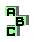POINT X(186)

 d point X(265) r304 circumcenter r584 orthocenter, point X(403), point X(468) r711 ninecircle, orthocentroidal circle, point X(24), inverse points r714 ninecenter, circumcenter, circumcircle, far-out point, inverse points r772 orthic triangle r1020 circumcevian triangle, centroid, orthocenter, circumcenter, point X(858) r1101 orthoassociate points, ninecenter r1218 ninecircle, cevian triangle, point X(403) r1241 Apollonian circles r1401 Jerabek hyperbola, point X(74), Kosnita point r1402 Jerabek hyperbola, point X(265), ninecenter r1517 circumconic, isodynamic points r1672 orthocenter, Euler line, altitude feet, circumcevian triangle r1971 orthocenter, circumcenter, isogonal points, tripolar, point X(275) r1983 circumcenter, ninecenter, altitude feet, Euler line